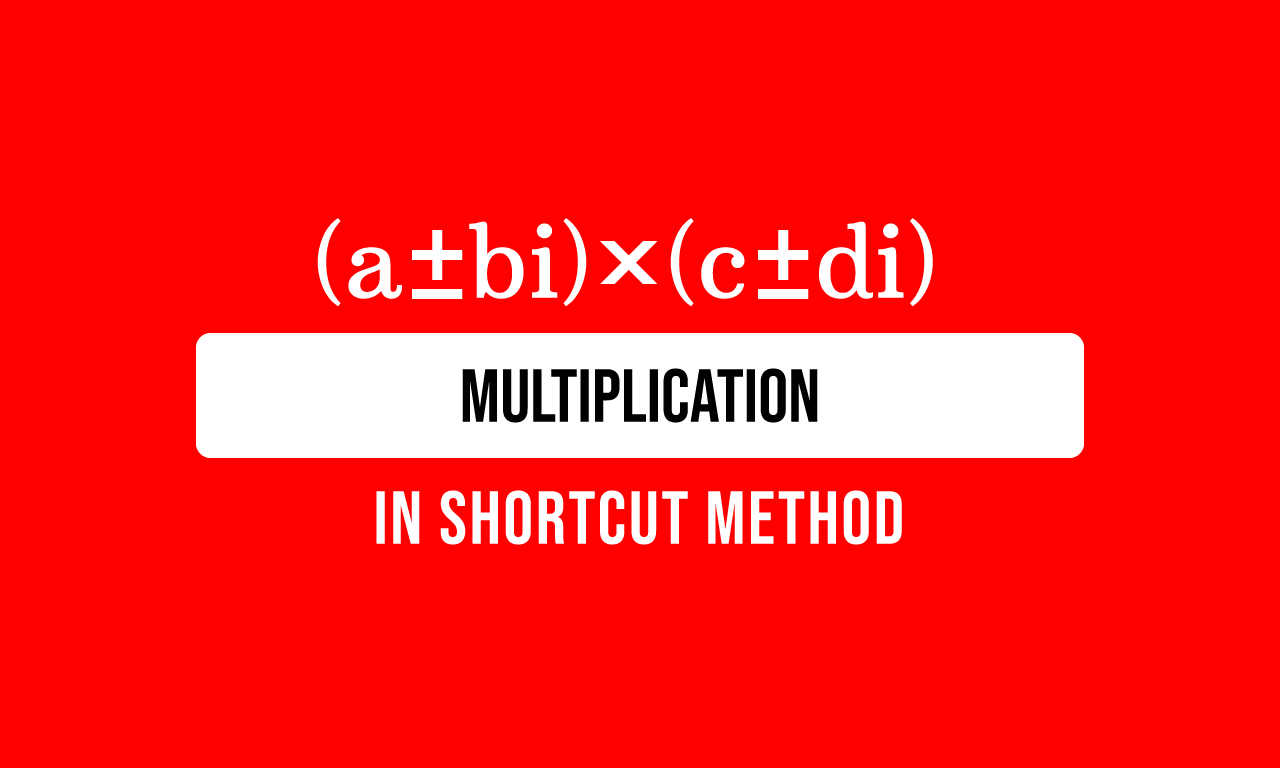# How to Multiply the Complex numbers in shortcut method

The multiplication of the complex numbers can also be calculated in a shortcut method to find the product of them. The following three fundamental concepts are used in this method. Therefore, learn them firstly before start learning the procedure for multiplying the complex numbers.The multiplication of the complex numbers is written in mathematics as follows.

$(a+bi)$ $\times$ $(c+di)$

The multiplication of the complex numbers is simply written in product form as follows.

$=\,\,\,$ $(a+bi)(c+di)$

Now, let’s learn how to multiply two or more complex numbers easily by the above mathematical concepts.

### Multiply the complex number by each term

$(a+bi)$ $\times$ $(c+di)$

$a$ and $bi$ are the terms in one complex number, and $c+di$ is another complex number in the expression. Now, multiply the complex number $c+di$ by each term of another complex number.

$=\,\,\,$ $a$ $\times$ $(c+di)$ $+$ $bi$ $\times$ $(c+di)$

Remember, the signs of the terms of complex number should be considered while multiplying another complex number.

### Multiply the terms by their coefficient

$a$ is a coefficient of the complex number $c+di$ in the first term and $bi$ is a coefficient of the complex number $c+di$ in the second term. Now multiply the factors in each term as per the distributive property.

$=\,\,\,$ $a \times c$ $+$ $a \times di$ $+$ $bi \times c$ $+$ $bi \times di$

### Simplify the mathematical expression

The process of multiplying the complex numbers is completed and it forms the mathematical expression. Now, it is time to multiply the factors in every term of the mathematical expression.

$=\,\,\,$ $ac$ $+$ $adi$ $+$ $bic$ $+$ $bdi^2$

$=\,\,\,$ $ac$ $+$ $adi$ $+$ $bic$ $+$ $bd \times i^2$

According to the complex numbers, the square of the imaginary unit is minus one. So, replace the square of the imaginary unit by its value in the fourth term of the above expression.

$=\,\,\,$ $ac$ $+$ $adi$ $+$ $bic$ $+$ $bd \times (-1)$

$=\,\,\,$ $ac$ $+$ $adi$ $+$ $bic$ $-$ $bd$

According to the commutative property, let’s write the terms and then the factors in each term in a convenient order for expressing the mathematical expression in a simplified form.

$=\,\,\,$ $ac$ $-$ $bd$ $+$ $adi$ $+$ $bic$

$=\,\,\,$ $ac$ $-$ $bd$ $+$ $adi$ $+$ $bci$

$=\,\,\,$ $ac$ $-$ $bd$ $+$ $bci$ $+$ $adi$

The imaginary unit is a common factor in both third and fourth terms. So, it can be taken common from them by taking the common factor out from the terms.

$=\,\,\,$ $(ac-bd)$ $+$ $(bc+ad)i$

$\therefore\,\,\,$ $(a+bi)(c+di)$ $\,=\,$ $(ac-bd)$ $+$ $(bc+ad)i$

Latest Math Topics
Jun 26, 2023
Jun 23, 2023

Latest Math Problems
Jul 01, 2023
Jun 25, 2023
###### Math Questions

The math problems with solutions to learn how to solve a problem.

Learn solutions

Practice now

###### Math Videos

The math videos tutorials with visual graphics to learn every concept.

Watch now

###### Subscribe us

Get the latest math updates from the Math Doubts by subscribing us.